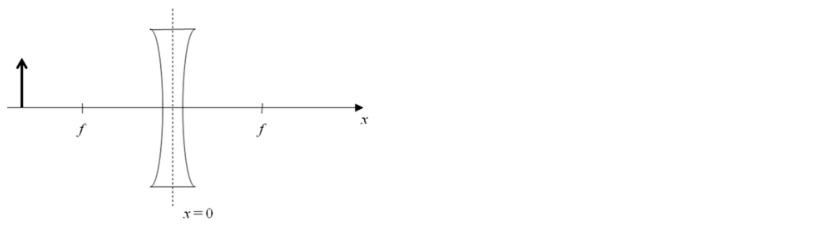# Problem: An object (bold arrow) is located at a distance of 3 f / 2 (i.e. at x = 3 f / 2) in front of a diverging lens as shown in the figure. Note that f is positive, so the focal length of this lens is - f. Suppose the object is now placed at x = - f. What is the magnification M? a) M = 0 b) M = 1/2 c) M = 1

###### FREE Expert Solution
82% (451 ratings)
###### Problem Details

An object (bold arrow) is located at a distance of 3 f / 2 (i.e. at x = 3 f / 2) in front of a diverging lens as shown in the figure. Note that f is positive, so the focal length of this lens is - f.

Suppose the object is now placed at x = - f. What is the magnification M?

a) M = 0

b) M = 1/2

c) M = 1